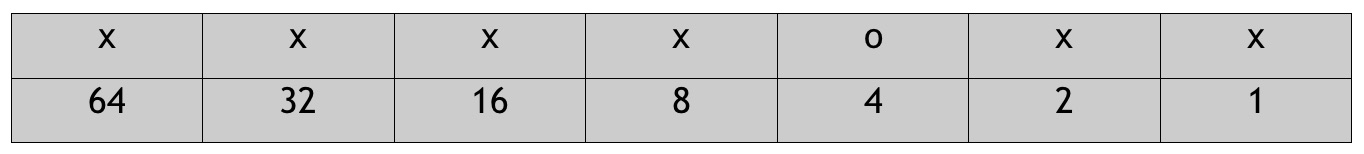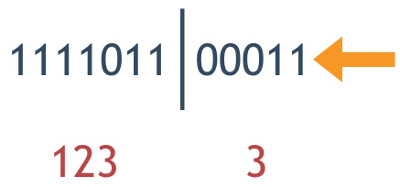## Wednesday, May 31, 2017

### Bit Shifting for a Shard ID in Ruby

As our database grew, we had to take a serious look at how can we could split it up by our clients, as most of them wanted to have their own data separate from the others anyway. A few months ago I found a great article from Pinterest, that describes how they sharded their MySQL database.

A sharded entity needs a UUID to uniquely identify the record across all shards. Most of the programming languages can generate a UUID easily, however, what was amazing to me, was that Pinterest generated its own unique ids by encapsulating three distinct numbers in one. Wait, what??! Read that article, it’s definitely worth your time.

While Pinterest encapsulated three numbers into one, we only needed two, a `client_id` and an `entity_id`. Our `client_id` would be a much smaller number than our `entity_id`, we wanted to reserve more bits for the latter.

It turns out, Ruby has many friendlier tools to deal with binary operations. Let's look at them!

What is the binary representation of the integer number 123?Out of the 7 bits, you'll see that the 3rd one is turned off, all the others are turned on, giving us 64 + 32 + 16 + 8 + 2 +1 = 123. How can we get this binary representation in Ruby? It's super easy, just use the to_s(2) method to do it.

```pry(main)> 123.to_s(2)
=>"1111011"
```

This is the exact same string representation as the one in the image above, where the third bit is turned off and represented with a zero.

I'd like to keep the `client_id` on the left side, but I'd like to reserve bits on the right side. For the sake of simplicity, I will keep this as a small number. Let's add 5 bits to the right-hand side of these bits by using the bitwise left shift operator.

```pry(main)> (123 << 5).to_s(2)
=> "111101100000"
```

The original number, 123, is still represented on the left side, but 5 "0"s were added to the right-hand side. You get the numeric representation of this by leaving out the `to_s(2)` call at the end:

```pry(main)> 123 << 5
=> 3936
```

This number can be converted back to binary:

```pry(main)> 3936.to_s(2)
=> "111101100000"
```

On the left side I have the binary representation of 123, but how about those bits on the right side? What are those representing? Right now, those bits are all turned off, they will give you 0 (`"00000".to_i(2) => 0`). How can I store the number 3 on the right side? The bits should look like this:The binary "|" will turn the two rightmost bits on:

```pry(main)> (123 MM 5 | 3).to_s(2)
=> "111101100011"
```

Again, leaving out the `to_s(2)` will provide the number representation:

```pry(main)> (123 << 5 | 3)
=> 3939
```

The storing part will work, but how can we get our two numbers back from this one combined number? Well, we have to split the bits and convert the binary representation to an integer.

Five bits were used on the right side to store our second number. We need to chop those off to get the number stored on the left side. The bitwise right shift (>>) will do just that:

```pry(main)> (3939 >> 5).to_s(2)
=> "1111011"
```

The string "1111011" is our original 123 in a binary string format. We can convert that to an integer by using the to_i(2) String method:

```pry(main)> (3939 >> 5).to_s(2).to_i(2)
=> 123
```

I right shifted the original number, 3939, converted it to a binary string and converted that to an Integer.

There are more efficient ways to do this by using a binary "&" `(3939 >> 5) & 0b1111111 => 123` with the max value the bits can represent. That's what the Pinterest article had, but I found using the Ruby conversion methods a bit more friendly to those of us who are not dealing with binary data on a daily basis.

We have the number on the left side, but what about the number on the right side? When we convert the number representation (3939) to a binary string, we know that the five characters on the right side will represent the bits of our other number. Ruby String’s `last(x)` will do just that:

```pry(main)> (3939 >> 0).to_s(2).last(5)
=> "00011"
```

Converting this binary String to Integer should be similar to what we've done before:

```pry(main)> (3939 >> 0).to_s(2).last(5).to_i(2)
=> 3
```

Using the binary "&" with the max number the bits can store will do this conversion in one step: `(3939 >> 0) & 0b11111 => 3`. As a side note, the binary number can be represented as a hexadecimal value: `(3939 >> 0) & 0x1F => 3`. This is a lot shorter than a series of ones and zeros.

There is a limit of how large the numbers can be as you have a limit of bits to store those. The max number can be determined by flipping the available bits on. For an 7 bit number it's `64 + 32 + 16 + 8 + 4 + 2 + 1 = 127` or `2**x-1`, where x is the number of bits. In our case it is `2**7-1 = 127`.

We ended up using a 64-bit Integer for our `shard_id`, which is a BIGINT in MySQL. We store `client_id` in 22 bits giving us the maximum of `2**22-1 = 4_194_304` and 40 bits for the `entity_id` with `2**40-1 = 1_099_511_627_775` max value. The remaining two bits are "worth in gold, we can expand the number or store a third (albeit small) number in it.